# Light Ray Path

## Homework Statement

The diagrams below illustrate the formation of a rainbow. Figure 1 shows the general arrangement and Figure 2 shows the path of a ray through a raindrop with the centre of the raindrop is labelled O.

Figure 1: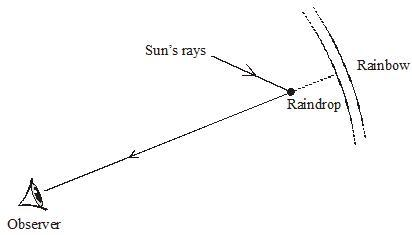Figure 2: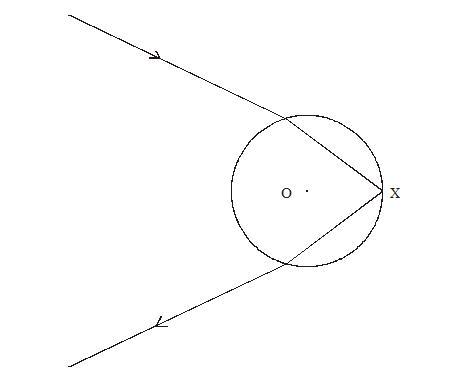a.)
Where the ray enters the raindrop in Figure 2, mark the angle of incidence i and the angle of refraction, r.

b.)
Figure 2 is drawn to scale. By taking suitable measurements, show that the refractive index of water is about 1.3.

## Homework Equations

$\mu = \frac{\sin i}{\sin r}$

## The Attempt at a Solution

a.)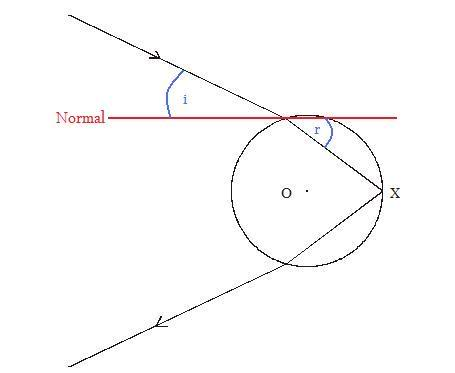^ Have I labelled it correct?

b.)
I measured the angle that I have labelled above and got $i = 25^\circ$ and $r = 35^\circ$ and this gives $\mu = 0.74$. This is incorrect. The correct answer is $i = 53, \ r = 39, \ \implies \mu = 1.27$. Where have I gone wrong here?

Redbelly98
Staff Emeritus
Homework Helper
The normal should be perpendicular to the water's surface.

So, is it like this?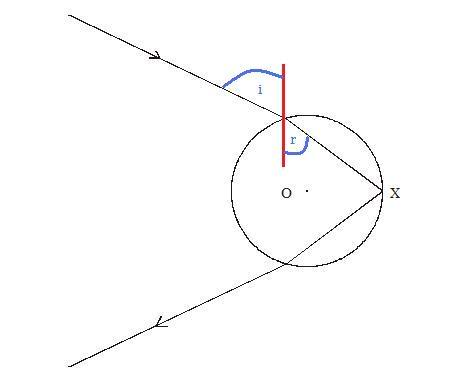Or, is it like this?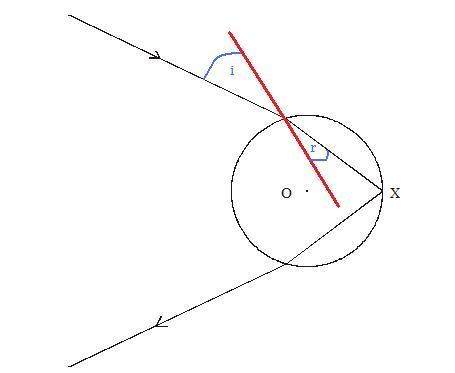You just seem to be drawing random lines lol...

The normal line on a spherical surface at some point P is always the line that goes from P through the center of the circle.

In other words; if you draw a straight line through the center of a circle, then at the points where it intersects the circle the angle between the line and the circle (at that point) is exactly 90 degrees, or perpendicular.

You just seem to be drawing random lines lol...

The normal line on a spherical surface at some point P is always the line that goes from P through the center of the circle.

In other words; if you draw a straight line through the center of a circle, then at the points where it intersects the circle the angle between the line and the circle (at that point) is exactly 90 degrees, or perpendicular.

Oooh, I understand now. I guess it is this: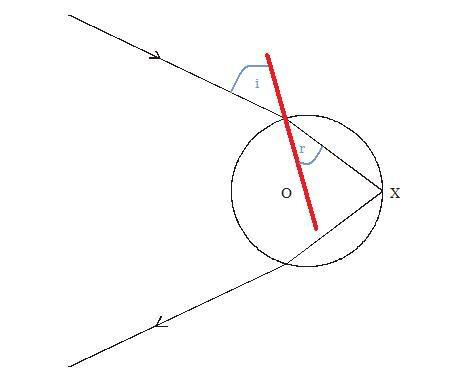Am I correct?

Last edited:
Redbelly98
Staff Emeritus